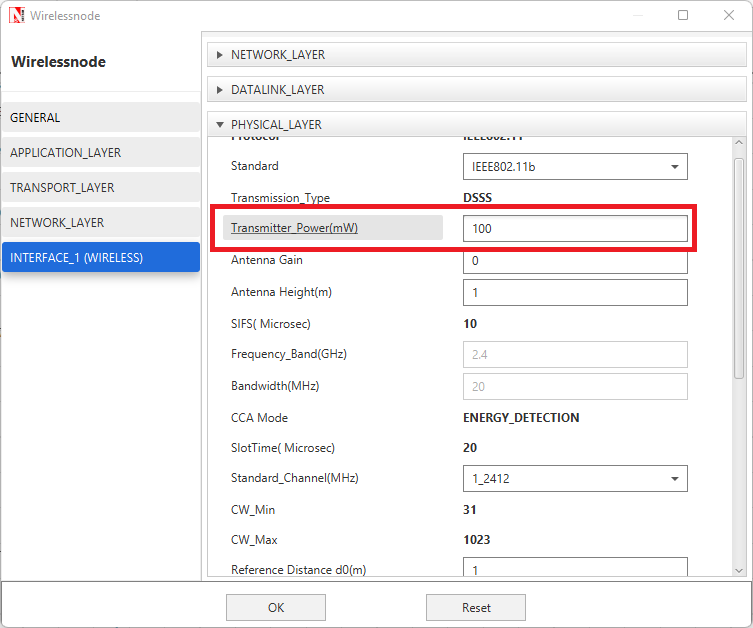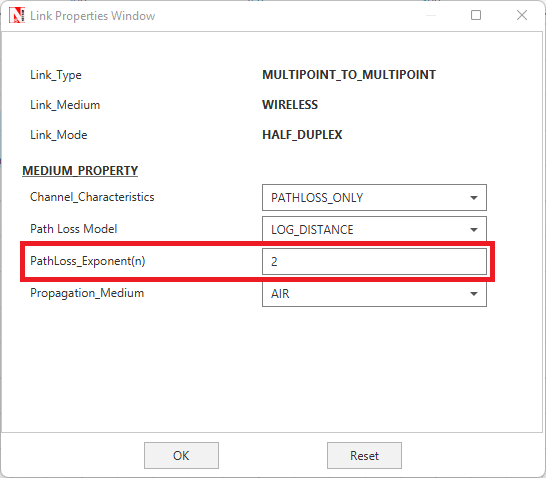The radio range of a node in NetSim is affected by the following factors:

a. Transmitter power (More Tx power implies higher range)
b. Path loss (Higher path loss exponent leads to the lower range)
c. Receiver sensitivity (Lower Rx sensitivity leads to a higher range. Rx sensitivity is in negative dB, hence lower means more negative).
d.  Distance between the nodes will have an impact on the received signal strength since path loss increases with distance.

Pathloss from the standard pathloss equation is equated to the receiver sensitivity to compute the radio range, viz.,where TX(power)  is the transmitter Power,
G(T) is the transmit antenna gain,
PL(do) is the reference distance path loss,
η  is the path loss exponent,
d(T) is the transmitter range
d(0) is the reference distance

The reference distance path loss (PL(d0)) is given by,**

We now consider an example involving IoT/WSN which uses the 802.15.4 standard.
In 802.15.4,d(0) the reference distance is 8m, while the operating frequency is 2400MHz. Applying these in the above formula, we get PL(do)  58 dB.
Given a transmitter power of 1mW or 0dBm, zero gains for the transmit and receive antennas, and, we can compute the transmit range, as follows:The transmission range of the sensor and 6lowpan gateway is 177.04m in the default setting of sensor module in NetSim.

**

The factors which affect the transmission range are:

a. Transmitter power (More Tx power leads to higher range)b. Path loss (Higher path loss exponent leads to a lower range)c. Receiver sensitivity (Lower Rx sensitivity leads to a higher range. Rx sensitivity is in negative dB, hence lower means more negative).

Note

1. There is a common misunderstanding that a range of 100 m implies that transmissions work perfectly till 99 m but suddenly stop at 100 m. This is not so. The error rate gradually increases as the range in increased if other parameters are kept constant.

The criteria for multi-hop communication is that MANET Route Request (or) Reply should not reach directly but should reach when sent via an intermediate node. The probability of RREQ or RREP being error-ed based on radio range is very less since these packets are very small. (PER is proportional to packet length).